# E-PolyLearning

 6. The equivalent diameter for flow through a rectangular duct of width B and height H is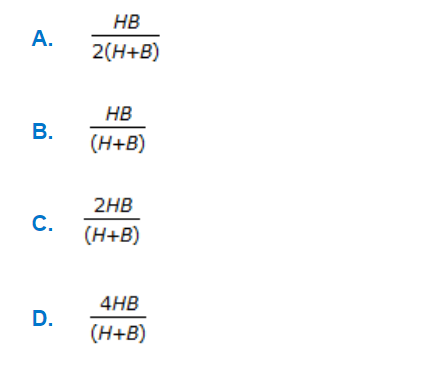a. A b. B c. C d. D

 7. Pick out the Hagen-Poiseulle's equation.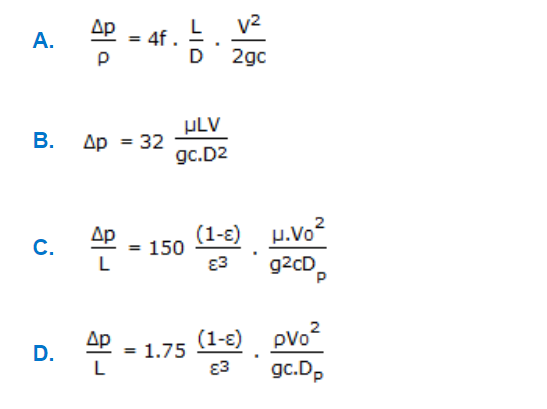a. A b. B c. C d. D
 8. During fluid flow, variation of shear stress(τ) with velocity gradientat (dv/dy) constant pressure temperature is shown below in the figure. In the below figure, Binghom plastic is represented by the curve .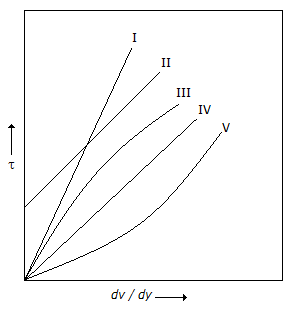a. V b. II c. III d. I
 9. Which is the correct relationship for a centrifugal pump ? (where, D = Impeller diameter, inches H = Head developed, ft of liquid pumped N = Speed of pump, rpm)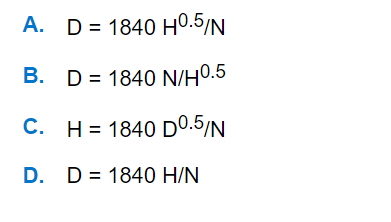a. A b. B c. C d. D
 10. The location of centre of pressure, which defines the point of application of the total pressure force on the surface, can be calculated by applying the principle of moments according to which "sum of the moment of the resultant force about an axis is equal to the sum of the components about the same axis". The centre of pressure of a rectangular surface (of width 'w') immersed vertically in a static mass of fluid is at a depth of(where, y = depth of the liquid)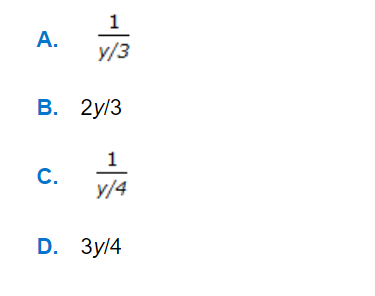a. A b. B c. C d. D